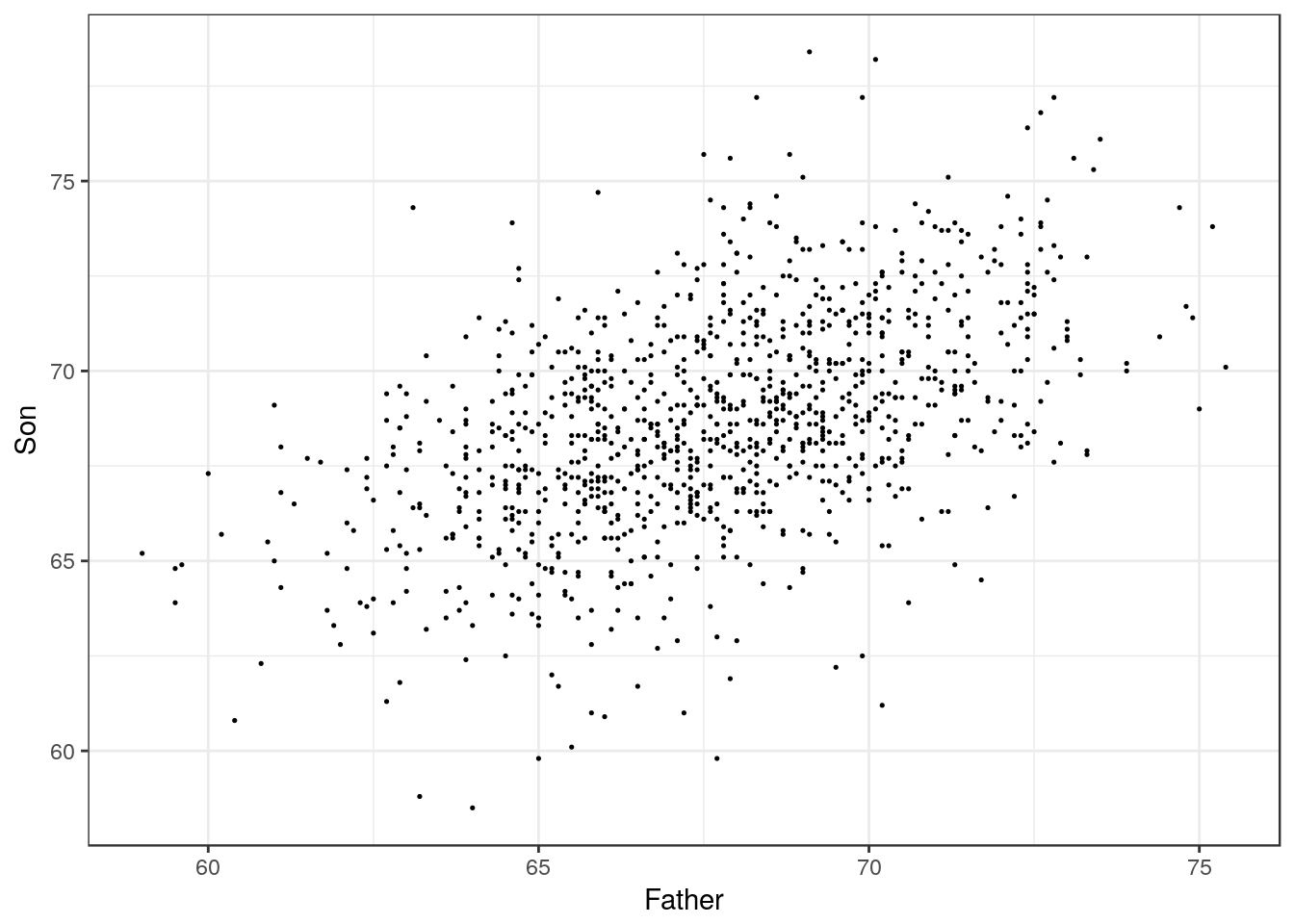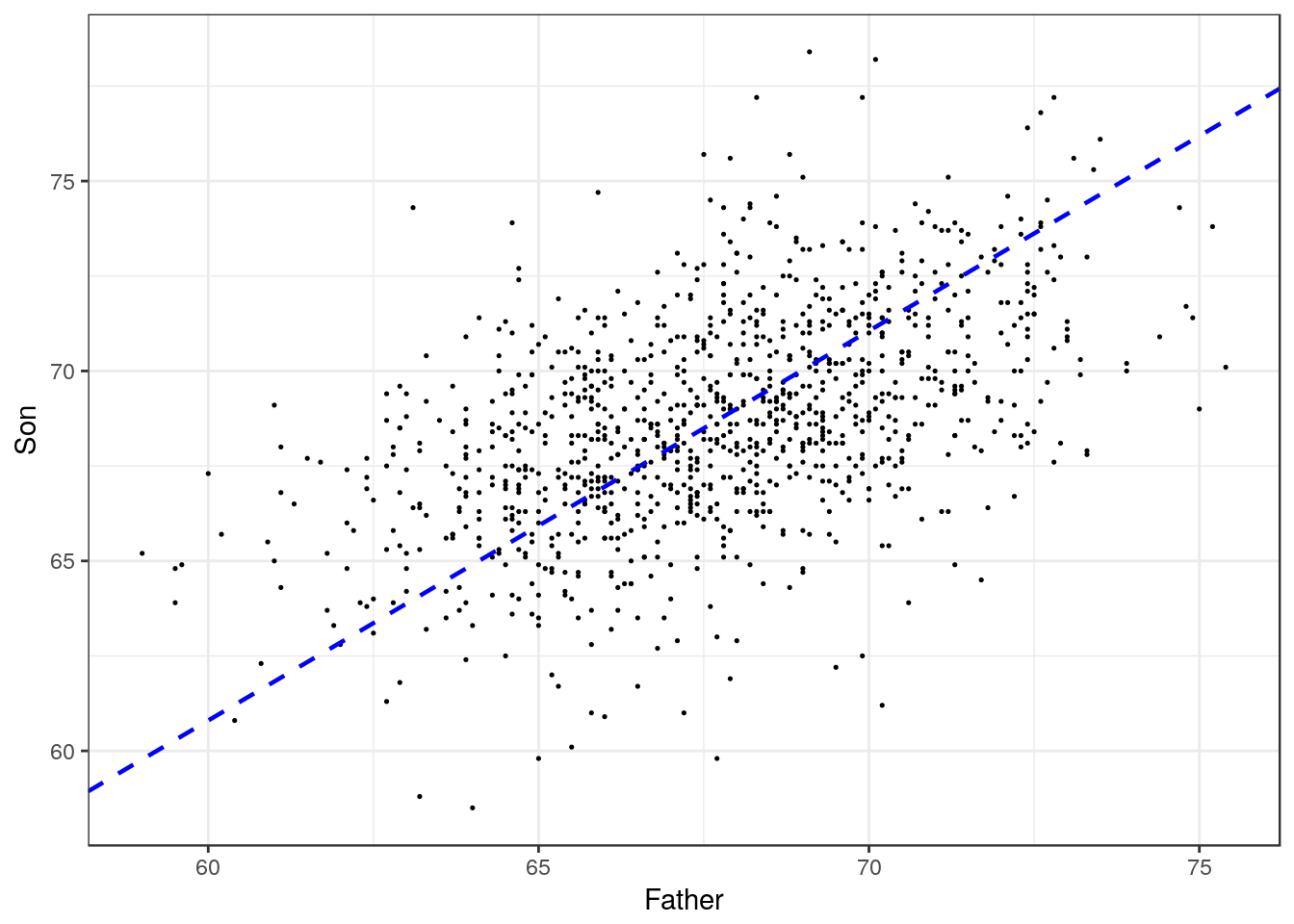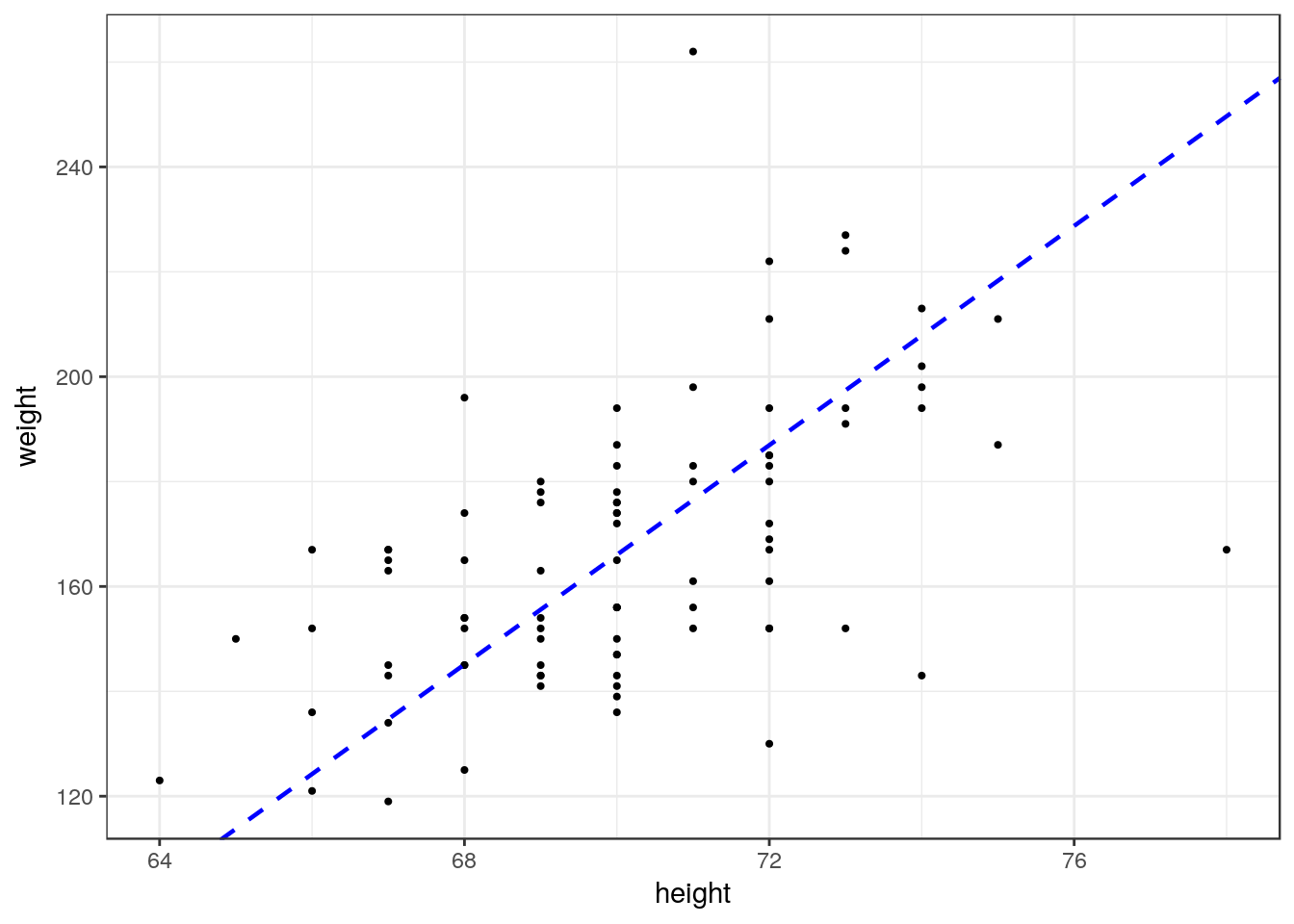## Heights of fathers and sons

``````father_son <- read_tsv("father_son.tsv")
``````## # A tibble: 6 × 2
##   Father   Son
##    <dbl> <dbl>
## 1   65.0  59.8
## 2   63.3  63.2
## 3   65.0  63.3
## 4   65.8  62.8
## 5   61.1  64.3
## 6   63.0  64.2``````

``````qplot(data = father_son,
x = Father, y = Son, geom = "point", size=I(0.25))``````## The standard deviation line

``````sd_line <- function(x, y) {
slope <- sd(y)/sd(x)
intercept <- mean(y) - mean(x) * slope
geom_abline(slope = slope, intercept = intercept,
linetype = "dashed", color = "blue", size=0.8)
}``````

``````qplot(data = father_son,
x = Father, y = Son, geom = "point", size=I(0.25)) +
sd_line(father_son\$Father, father_son\$Son)````````````measures <- read_tsv("heights_weights.tsv")
``````## # A tibble: 6 × 2
##   weight height
##    <int>  <int>
## 1    169     72
## 2    150     70
## 3    167     67
## 4    167     66
## 5    152     73
## 6    156     70``````

``````p <- qplot(data = measures, x = height, y = weight,
geom = "point", size=I(0.75)) +
sd_line(measures\$height, measures\$weight)
p````````````p +
geom_point(aes(x = mean(height) + sd(height),
y = mean(weight) + sd(weight)),
color = "blue", size = 2) +
geom_point(aes(x = mean(height) - sd(height),
y = mean(weight) - sd(weight)),
color = "blue", size = 2)``````